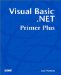# While End While Loops

### While End While Loops

The general syntax structure for a While loop is

`  While   Expression1   WhileStatementBlock   End While  `

The While statement is based on the Boolean evaluation of Expression1 . As long as Expression1 evaluates to logic True , the program statements in WhileStatementBlock are executed. If there are multiple statements in the While statement block, control is sent back to Expression1 for reevaluation after the last statement is executed. When Expression1 evaluates to logic False , program control is sent to whatever statement follows the End While keywords.

#### While End While Syntax Rules

Let's explore this syntax with the following code fragment:

` Dim Number as Integer  Number = 10 While Number < 20   DoThis()   Number += 1 End While `

Notice that the While loop executes as long as Number is less than 20. Expression1 , therefore, is a relational comparison that must yield a Boolean result of either True or False. The fact that Expression1 is a Boolean result has two important implications.

First, it's possible for the statements controlled by the While loop never to be executed. For example, suppose that we assigned 30, instead of 10, into Number in the preceding code fragment. In that case, the Boolean result for Expression1 (that is, Number < 20 ) would be False when it's first evaluated. This means the two statements controlled by the While would never be executed.

Second, if Expression1 evaluates to True , the statements controlled by the While are executed. Equally important, however, is that some statement in the While statement block must be able to falsify the outcome of Expression1 at some point. If the statements cannot falsify Expression1 , an infinite loop results. For example, what would happen if we removed the statement

` Number += 1 `

from the code fragment? In that situation, Expression1 would always be True because no statement in the loop alters the initial value of Number . This results in an infinite loop, which would cause the program to hang in the While loop. Not good.

It should be clear from this discussion that well behaved While loops require the same three conditions we studied in Chapter 12. That is, the variable that's used to control the loop must be initialized , the variable needs to be evaluated by a relational test, and the variable must be able to assume a value that will result in a logic False evaluation.Visual Basic .NET Primer Plus
ISBN: 0672324857
EAN: 2147483647
Year: 2003
Pages: 238
Authors: Jack Purdum

Similar book on Amazon